Next: 2-d problem with Dirichlet Up: The diffusion equation Previous: An improved 1-d diffusion

## An improved 1-d solution of the diffusion equation

Let us now solve the simple diffusion problem introduced in Sect. 6.4 with the above listed Crank-Nicholson routine. Figure 73 shows a comparison between the analytic and numerical solutions for a calculation performed using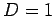,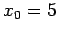,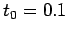,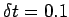, and. It can be seen that the analytic and numerical solutions are in excellent agreement. Note, however, that the time-step used in this calculation (i.e.,) is much larger than that used in our previous calculation (i.e.,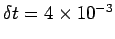), which employed an explicit differencing scheme--see Fig. 71. According to Eq. (209), an explicit scheme is limited to time-steps less than about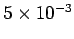for the problem under investigation. Thus, we have been able to exceed this limit by a factor of 20 with our implicit scheme, yet still maintain numerical stability. Note that our Crank-Nicholson scheme is able to obtain accurate results with a time-step as large as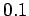because it is second-order in time.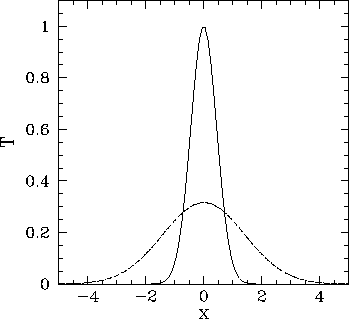Next: 2-d problem with Dirichlet Up: The diffusion equation Previous: An improved 1-d diffusion
Richard Fitzpatrick 2006-03-29